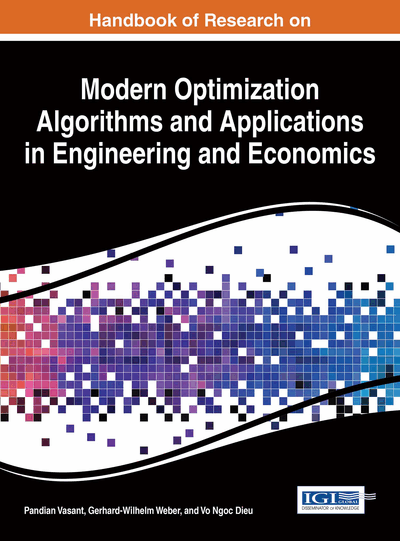# Imprecise Solutions of Ordinary Differential Equations for Boundary Value Problems Using Metaheuristic Algorithms

Ali Sadollah (Korea University, South Korea) and Joong Hoon Kim (Korea University, South Korea)
Copyright: © 2016 |Pages: 21
DOI: 10.4018/978-1-4666-9644-0.ch015
OnDemand PDF Download:
Available
\$37.50
No Current Special Offers

## Abstract

In this chapter, a general strategy is recommended to solve variety of linear and nonlinear ordinary differential equations (ODEs) with boundary value conditions. With the aid of certain fundamental concepts of mathematics, Fourier series expansion, and metaheuristic algorithms, ODEs can be represented as an optimization problem. The purpose is to reduce the weighted residual error (error function) of the ODEs. Boundary values of ODEs are considered as constraints for the optimization model. Inverted generational distance metric is utilized for evaluation and assessment of approximate solutions versus exact solutions. Four ODEs having different orders and features are approximately solved and compared with their exact solutions. The optimization task is carried out using different optimizers including the particle swarm optimization and the water cycle algorithm. The optimization results obtained show that the proposed method equipped with metaheuristic algorithms can be successfully applied for approximate solving of different types of ODEs.
Chapter Preview
Top

## Introduction

Mathematical formulation of most physical and engineering problems involves differential equations (DEs). DEs have applications in all areas of science and engineering. Hence, it is important for engineers and scientists to know how to set up DEs and solve them. With regard to real life problems, which are highly nonlinear, many problems in engineering often include one or more ordinary DEs (ODEs).

Analytical approaches are often incompetent in tackling ODEs (except the simple ODEs). Therefore, approximate analytical methods are applied to obtain solutions of ODEs. A number of analytical methods have been used to obtain approximate analytical solutions for engineering problems, such as the variational iteration method (Coşkun & Atay, 2008), the homotopy analysis method (Domairry & Fazeli, 2009), and differential transformation method (Zhou, 1986).

In particular, many studies have applied approximation methods for solving various types of integro-differential equations (linear/nonlinear) (Darania & Ebadian, 2007; Darania & Ivaz, 2008; Roul & Meyer, 2011). However, each of these numerical approximation techniques has its own operational limitations that strictly narrow its functioning domain. Hence, it is possible that these approximate techniques fail to overcome a specific problem (Torabi et al., 2012; Yaghoobi & Torabi, 2012).

Despite there being a wide range of approximate methods for solving ODEs, there is a lack of a general approach that meets all the engineering demands having unconventional and nonlinear ODEs. It should be very interesting to solve linear and nonlinear ODEs having arbitrary boundaries values.

Metaheuristic algorithms are usually devised by observing the phenomena occurring in nature. With the emergence of metaheuristic algorithms, complex problems having large dimensions may optimally solve. Metaheuristic optimization algorithms have demonstrated their efficiency in finding near-optimal solutions where exact and analytical approaches may not be able to produce superior solutions within reasonable computational time (Glover & Kochenberger, 2003; Yang, 2010).

Up to date, applications that use metaheuristic algorithms for finding estimated solution of ODEs have significantly increased. For instance, the genetic algorithms (Mastorakis, 2006), the particle swarm optimization (PSO) (Lee, 2006; Babaei, 2013), the genetic programming (Cao et al., 2000). However, the strategies applied in these methods are different with each other.

Recently, the concept of Fourier series expansion has been used as a base approximate function for finding the approximate solution of ODEs. Hence, the ODEs problem is modeled as an optimization problem and solved using the PSO (Babaei, 2013). For simplicity, the weight function considered in the literature was set to unit weight function (i.e., constant weigh function equal to one) (Babaei, 2013). However, this assumption may not help us in obtaining better results for different types of ODEs. Recently, Sadollah et al. (2015) has shown superiority of a new proposed weight function for several ODEs and mechanical problems and compared with the unit weight function.

In this paper, using the concept of Fourier series as the base approximate function, other optimizers, including the water cycle algorithm (WCA) (Eskandar et al., 2012) and the PSO (Kennedy & Eberhart, 1995) are applied for optimization purposes. Furthermore, new weight function called least square weight function is applied instead of unit weight function in this chapter. The WCA, recently used metaheuristic algorithms, is inspired by observation of water cycle process particularly surface runoff. Both the PSO and WCA have been implemented in optimization phase in this chapter.

## Key Terms in this Chapter

Water Cycle Algorithm: A population-based optimization method inspired by the water cycle process and observation of how rivers and streams flow to sea.

Approximate Solution: Sometimes it is difficult to solve an equation exactly. However, an approximate solution may be accurate enough for solving the considered equation.

Fourier Series: A Fourier series is an expansion of a periodic function f(x) in terms of an infinite sum of sines and cosines. The computation and study of Fourier series is known as harmonic analysis and is extremely useful as a way to break up an arbitrary periodic function into a set of simple terms.

Metaheuristic Algorithms: A metaheuristic is defined as an iterative approach which guides a subordinate heuristic by combining intelligently different concepts for exploring and exploiting search space. They are inspired by observing the phenomena occurring in nature.

Ordinary Differential Equations: An ordinary differential equation (ODE) is an equality involving a function and its derivatives.

Particle Swarm Optimization: A population-based optimization algorithm inspired by the swarm movements of flocks (e.g., fish, birds).

Inverted Generational Distance: The IGD is an assessment criterion, used for calculating Euclidean distance between exact and apprxixmate solutions.

Bundary Value Problems: A solution to a boundary value problem is a solution to the differential equation which also satisfies the boundary conditions.

## Complete Chapter List

Search this Book:
Reset